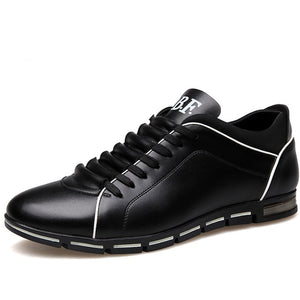# 2020 spring/summer men fashion lace-up leather shoes

• \$46.99 USD
Precio unitario por
• Ahorra \$32.96 USD

Size Note：

Choose your size according to your feet length, and we will be send the corresponding Chinese size. US size 5.5 =CN size 38=24.0 CM, US size 6 =CN size 39=24.5 CM, US size 6.5 =CN size 40=24.8 CM, US size 7 =CN size 40=25.0 CM, US size 7.5 =CN size 41=25.5 CM, US size 8 =CN size 42=25.8 CM, US size 8.5 =CN size 42=26.0 CM, US size 9 =CN size 43=26.5 CM, US size 10 =CN size 44=27.0 CM, US size 11 =CN size 45=27.5 CM, US size 12 =CN size 46=28.0 CM, US size 13 =CN size 47=28.5 CM, US size 14 =CN size 48=29.0 CM,